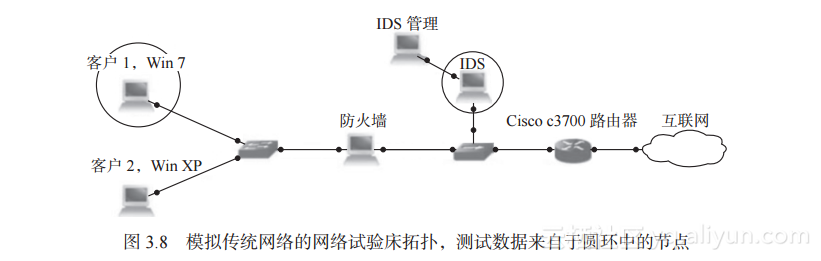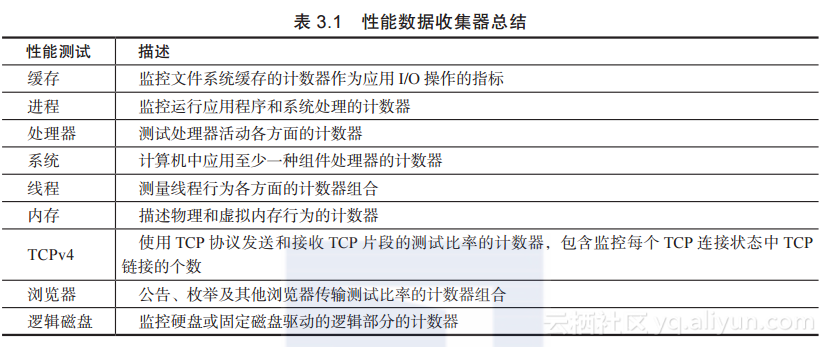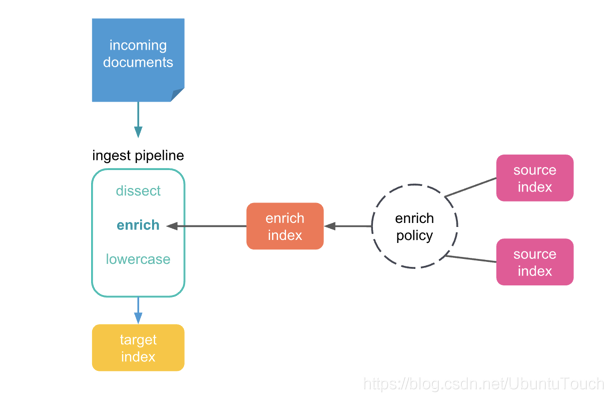# 《网络空间欺骗：构筑欺骗防御的科学基石》一3.3.3　现场分析

+关注继续查看

3.3.3　现场分析[PeterDLax著泛函分析习题参考解答]第6章 Hilbert 空间
1. 证明满足 (6) 的范数可以由一个内积诱导出来. 这个结论属于 von Neumann.   证明: 以实线性空间为例, 取内积 $$\bex \sex{x,y}=\cfrac{1}{4}[\sen{x+y}^2-\sen{x-y}^2], \eex$$ 则 $\sex{x,y}$ 为内积, 且 $\sex{x,x}^\frac{1}{2}=\sen{x}$.
654 0MaxCompute Studio使用心得系列3——可视化分析作业运行

3571 01480 0[PeterDLax著泛函分析习题参考解答]第1章 线性空间
1. 证明定理 1.     2. 验证上述结论.     3. 证明定理 3.     4. 证明定理 4.   证明: 由 $$\bex x=\sum_{k=1}^{n-1}a_k\cdot \sum_{j=1}^{n-1}\cfrac{a_j}{\sum_{k=1}^{n-1}a_k}x_j+a_nx_n \eex$$ 及数学归纳法即知结论.
464 0Observability：使用 Elastic Stack 分析地理空间数据

3605 0802 0《Spark大数据分析实战》——3.1节SQL on Spark
2003 0《Spark大数据分析实战》——2.3节Spark编译
1303 01389 0《网络空间欺骗：构筑欺骗防御的科学基石》一1.3　恶意策略、技术和常识

1382 0
10059

0

+ 订阅

OceanBase 入门到实战教程